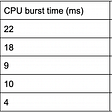# Find the Height of the Tree, Find the Diameter of the Tree

## Find the Height of the Tree

`class Node:    def __init__(self, data):        self.data = data        self.left = None        self.right = Nonedef height(node):    #if tree from the node not exist, return 0    if node is None:        return 0    # get the hight from the node's left tree and right tree     l_height = height(node.left)    r_height = height(node.right)    # find the longer one from left and right,    # then plus one for the current tree's maximum hight    return max(l_height, r_height) +1`

result

`root = Node(1)root.left = Node(2)root.right = Node(3)root.left.left = Node(4)root.left.right = Node(5)print('The height of the tree is {}'.format(height(root)))>>>>>>>>>>>>>>>>>>>>>>>>>>>>>>>The height of the tree is 3`

## Advanced: Find the Diameter of the Tree

To find a diameter of a tree, two situations could be the answer:
1. `left subtree's height + right subtree's height + 1(itself)`
2. `left subtree's diameter or right subtree's diameter (without itself)`

`class Node:    def __init__(self, data):        self.data = data        self.left = None        self.right = None        def height(node):    # Tree is empty    if node is None:        return 0    return max(height(node.left), height(node.right)) +1    def diameter(root):    # Tree is empty    if root is None:        return 0     # Get the height of left and right sub-trees    lheight = height(root.left)    rheight = height(root.right)     # Get the diameter of left and right sub-trees    ldiameter = diameter(root.left)    rdiameter = diameter(root.right)     # Return max of the following tree:    # 1) Diameter of left subtree    # 2) Diameter of right subtree    # 3) Height of left subtree + height of right subtree +1    return max(lheight + rheight + 1, ldiameter, rdiameter)`

result

`root = Node(1)root.left = Node(2)root.right = Node(3)root.left.left = Node(4)root.left.right = Node(5)print('The diameter of the tree is {}'.format(diameter(root)))>>>>>>>>>>>>>>>>>>>>>>>>>The diameter of the tree is 4`

--

--

--

## More from Coder Life

A place that share all value skills and techniques I learned in development.

## Exploring the Key Features of Laravel 7 Framework## Priority Based Round-Robin CPU Scheduling Algorithm with Case Study(Part-9)## A Higher Order Impact of Software Design## Quick-guide on Software Pre-Cert Program (6) — Summary## How I got into programming!## How You, The New Data Professional, Can Handle Your First Code Review## LFI in the ILIAS e-learning platform## Allie Hsu

a tech enthusiast who is keen to develop new skills

## Solving N-Queens for 1 Million Queens with MinConflict## Hurry up! Grab this great opportunity and get going…## Travelling Salesman Problem Using Simulated Annealing